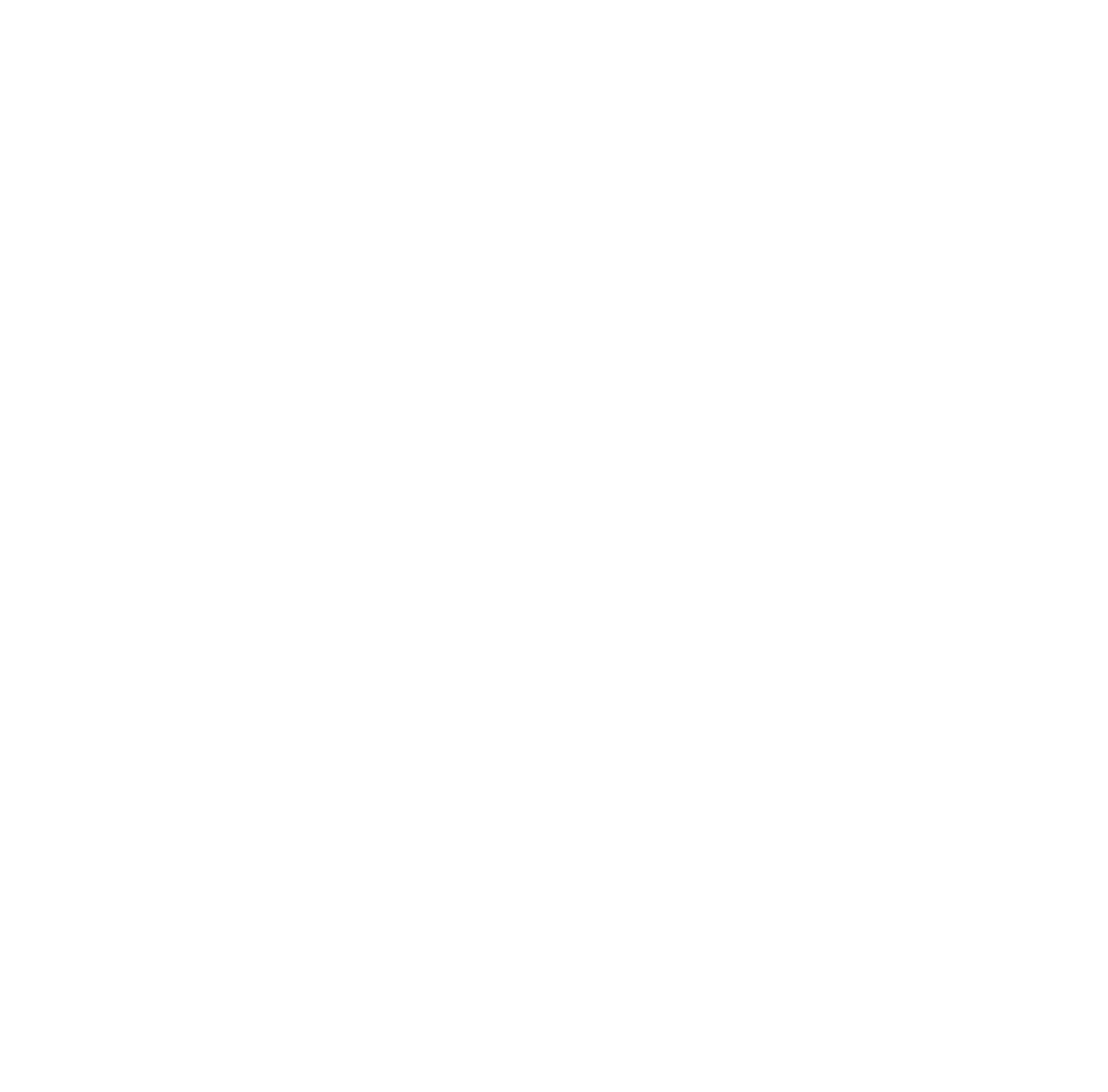# Districts of Serbia

Districts of Serbia. In Serbia there are a total of 29 (5 districts within the Kosovo borders) district. Districts form the first-level administrative divisions of Serbia. The capital Belgrade has a separate status. The 5 districts in Kosovo are not included in this article. On the map below you can see the districts of Serbia except Kosovo.

## Districts of SerbiaDistricts of Serbia

## Kolubara

It has a population of 174,228. The surface area is 2,474 square kilometers.

## Mačva

It has a population of 297,778. The surface area is 3,268 square kilometers.

## Moravica

It has a population of 212,149. The surface area is 3,016 square kilometers.

## Pomoravlje

It has a population of 212,839. The surface area is 2,614 square kilometers.

## Rasina

It has a population of 240,463. The surface area is 2,667 square kilometers.

## Raška

It has a population of 300,102. The surface area is 3,918 square kilometers.

It has a population of 290,900. The surface area is 2,387 square kilometers.

## Zlatibor

It has a population of 284,729. The surface area is 6,140 square kilometers.

## Bor

It has a population of 123,848. The surface area is 3,507 square kilometers.

## Braničevo

It has a population of 180,480. The surface area is 3,865 square kilometers.

## Jablanica

It has a population of 215,463. The surface area is 2,769 square kilometers.

## Nišava

It has a population of 373,404. The surface area is 2,729 square kilometers.

## Pčinja

It has a population of 158,717. The surface area is 3,520 square kilometers.

## Pirot

It has a population of 92,277. The surface area is 2,761 square kilometers.

## Podunavlje

It has a population of 198,184. The surface area is 1,248 square kilometers.

## Toplica

It has a population of 90,600. The surface area is 2,231 square kilometers.

## Zaječar

It has a population of 118,295. The surface area is 3,623 square kilometers.

## Central Banat

It has a population of 186,851. The surface area is 3,256 square kilometers.

## North Bačka

It has a population of 185,552. The surface area is 1,784 square kilometers.

## North Banat

It has a population of 146,690. The surface area is 2,329 square kilometers.

## South Bačka

It has a population of 615,371. The surface area is 4,016 square kilometers.

## South Banat

It has a population of 291,327. The surface area is 4,245 square kilometers.

## Srem

It has a population of 311,053. The surface area is 3,486 square kilometers.

## West Bačka

It has a population of 187,581. The surface area is 2,420 square kilometers.